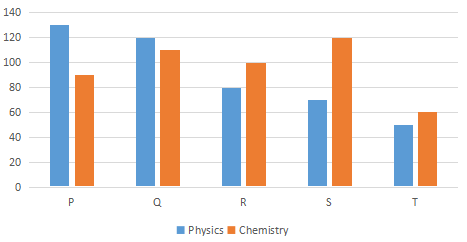## IBPS Clerk Quant Test 10

Instructions

Study the following bar graph carefully to answer the questions.Q 1

Marks obtained by S in Chemistry is what per cent of the total marks obtained by all the students in Chemistry ?

Q 2

If the marks obtained by T in Physics were increased by 14% of the original marks, what would be his new approximate percentage in Physics if the maximum marks In Physics were 140?

Q 3

Fill in the blank space in order to make the sentence correct as per the given information. Total marks obtained by T in both the subjects together is more than the marks obtained by -

Q 4

What is the respective ratio between the total marks obtained by P in Physics and Chemistry together to the total marks obtained by T in Physics and Chemistry together ?

Q 5

What is the respective ratio between the total marks obtained by Q and S together in Chemistry to the total marks obtained by P and R together in Physics ?